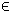## 21L1LIN Problem

The linearly constrained L1LIN (L1LIN) problem is defined as

 min x
|Cxy)| + alpha*|Lx|
subject to xL x xU
bL Ax bU
(23)
where x,xL,xURn, bL,bURm2, ARm1 × n, CRm2 × n, yRm2, LRn × b and alphaR1.

The L1Lin solution can be obtained by the use of any suitable linear TOMLAB solver.

The following file illustrates how to solve an L1Lin problem in TOMLAB.

File: tomlab/quickguide/L1LinQG.m

Open the file for viewing, and execute L1LinQG in Matlab.
```% L1LinQG is a small example problem for defining and solving
% a linearly constrained linear L1 problem using the TOMLAB format.

Name='L1LinSolve test example';      % Problem name, not required.
n = 6;
x_L = -10*ones(n,1);                 % Lower bounds on x
x_U =  10*ones(n,1);                 % Upper bounds on x
x_0 = (x_L + x_U) / 2;               % Starting point

C = spdiags([1 2 3 4 5 6]', 0, n, n); % C matrix
y = 1.5*ones(n,1);                    % Data vector

% Matrix defining linear constraints
A = [1 1 0 0 0 0];
b_L = 1;                  % Lower bounds on the linear inequalities
b_U = 1;                  % Upper bounds on the linear inequalities

% Defining damping matrix
Prob.LS.damp = 1;
Prob.LS.L = spdiags(ones(6,1)*0.01, 0, 6, 6);